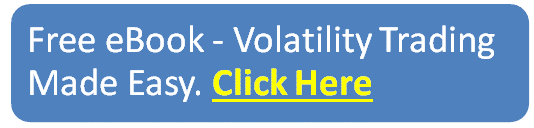blog

# The Ultimate Guide To Put Ratio Backspreads

#### Options Trading 101 - The Ultimate Beginners Guide To Options

As Seen On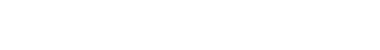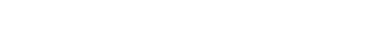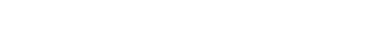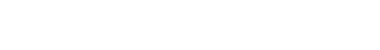by Gavin in Blog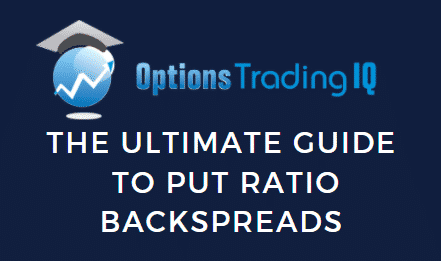## Contents

A put ratio backspread is an option trade that involves selling put options and buying a larger number of put options on the same instrument and the same expiration date.

The name of the trade comes from the ratio between the number of long and short positions.

The put ratio backspread (or reverse put ratio spread) is a bearish strategy that is created when the trader thinks that the stock will suffer a significant downside movement.

The most common ratio in put ratio backspreads is two-to-one, where there are twice as many long puts as short ones.

The strategy involves selling an in-the-money (ITM) put and buying two or more puts at a lower price, usually out-the-money (OTM), having the same expiration.

The strategy is usually initiated as for a net credit, this permits the trade to make a small gain even when the stock price moves higher.

The strategy is long vega and benefits from a rise in implied volatility.

There a is limited risk on the downside if the stock moves closer to the strike price of the long put (valley of death) or if the implied volatility decreases.

Some investors prefer this strategy over buying long puts because the trade can be initiated for a credit.

Here is an example of a BP put ratio backspread.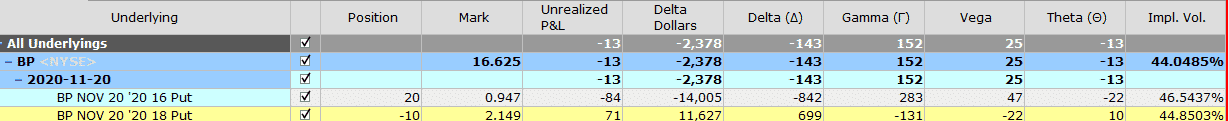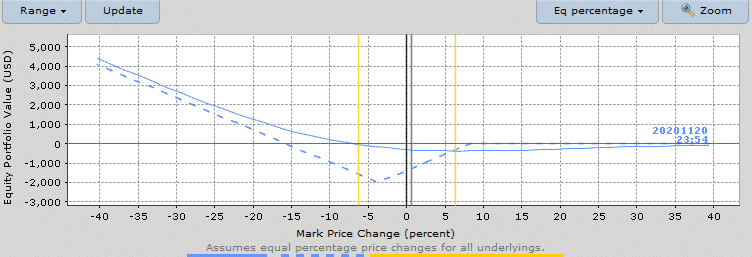## Maximum Loss

The maximum loss occurs when at expiry the stock is at the strike price of the long puts.

The maximum loss is equal to the intrinsic value of the short plus or minus the net debit or credit received when the trade was initiated.

In the BP case, there is no loss on the upside because the trade was created for a credit.

If the trade was created for credit, the maximum loss is:

Maximum loss = Strike price short put – strike price long put – Net credit received

Our BP put ratio backspread was initiated for a credit of \$255, the short BP 18 put, and long BP 16 pus are \$2 apart. The maximum loss of the trade would be:

Max loss = \$2000 – \$255 = \$1745

If the trade was initiated for a debit, the maximum loss is:

Maximum loss = Strike price short – strike price long puts + Net premium paid

## Maximum Gain

The maximum gain happens when the stock price drops to zero.

If this improbable but not impossible situation occurs, the short put would expire at the money as well as the long puts.

Max Gain = Long put price – (Short put price strike – Long put price strike) + Net credit

In our BP trade, the maximum gain is

10 × (\$16 – \$2 + \$0.255) x 100 = \$14,255

## Breakeven Price

If the put ratio backspread is established for a credit, there are two breakeven prices which are:

Upper breakeven price = strike price of the short put – net credit received

Lower breakeven price = strike price long put – (strike price short put – strike price long put) +  net credit received

In our BP example, the upper breakeven price is:

\$18 – \$0.255 = \$17.745

In our BP trade, the lower breakeven price is

\$16 – 2 + 0.255 = \$14.255

If the put ratio backspread is established for a debit, there is only one breakeven point:

Lower breakeven price = strike price of the long puts+ difference between the strikes – net debit paid

## Payoff Diagram

Put ratio backspreads have an inverted slanted tent-shaped payoff diagram.

This kind of shape results in high gamma near expiry. I also like to refer to the inverse profit tent as the “valley of death”.

It the trade is created for credit, put ratio backspreads have no risk on the upside.

The risk appears when the stock price stagnates between the two breakeven prices, that is, close to the long put strike.

For this reason, you initiate the trade when you expect a strong downside movement of the stock price.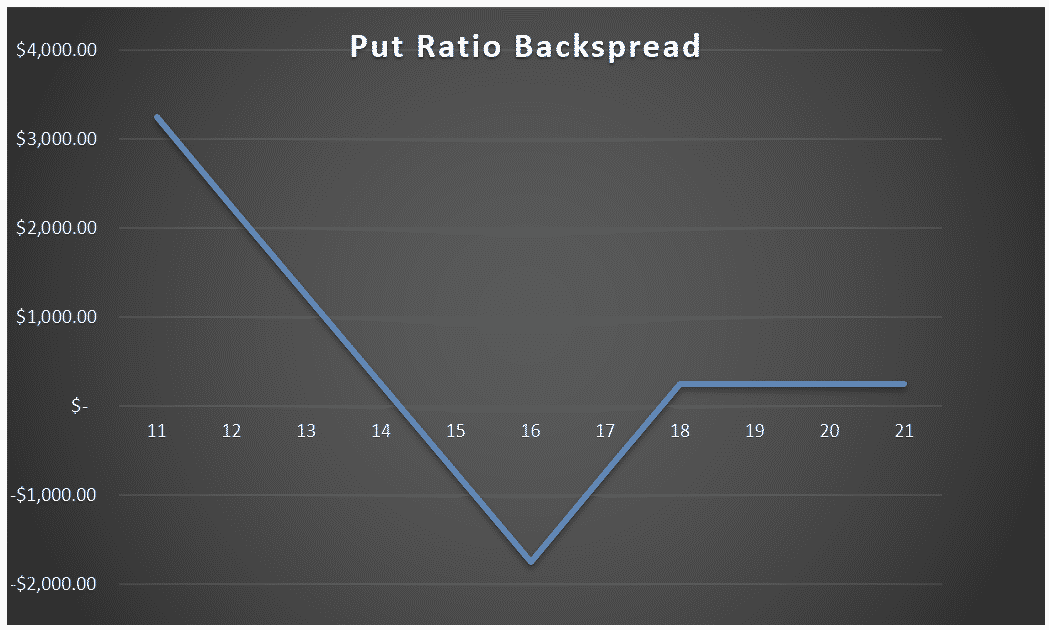## Risk of Early Assignment

There is always a risk of early assignment when the price of the underlying is below the strike price of the short put option.

Usually, an early assignment only occurs on put options when they are deep in the money, in this case, the short put

gets assigned, the position becomes a protective put plus a long put.

If the short put gets assigned and the trader can consider close the trade for a loss or keep the position until expiration if the stock was trading below the lower breaking price.

The trader should also consider the cost of having a long position in the underlying stock.

For this reason, it is advisable to close the trade one week before expiry date.

This is particularly relevant if the stock price is trading between the breakeven prices and the trade is close to the expiration date.

## How Volatility Impacts Put Ratio Backspreads

Put ratio backspreads are long vega trades, so generally speaking, they benefit from rising volatility after the trade has been placed.

Our BP example starts with a vega of 25. This means that for every 1% increase in implied volatility, the trade should gain \$25.

The opposite is true if implied volatility drops 1% – the position would lose \$25.

## How Theta Impacts Put Ratio Backpreads

Put ratio backspreads are positive theta when above the upper breakeven point and negative theta when below the upper breakeven point.

In our BP example, the trade has negative Theta of 13. This means that all else being equal, the trade will lose \$13 per day due to time decay.

Notice that the negative time decay on the long puts is higher than the time decay being suffered on the short puts.

If BP stock was above 17.75, the trade would switch to positive theta. Note that this only occurs if the trade is entered for a credit.

## Other Greeks

### Delta

Delta on a put ratio backspread can be positive or negative when the trade is initiated.

A positive delta trade is usually created selecting the short put with a higher strike price (ITM).

As the trade progresses, the delta will change, and it could change from positive to negative or vice-versa.

Our BP trade has an initial negative delta of 143. This means that all else being equal, for every \$1 drop in the price of BP, the trade will make \$143.

### Gamma

Put ratio backspreads are positive gamma trades, they benefit from changes in the delta of the position.

In other words, you want the stock to move, hopefully below the lower breakeven point.

In our BP example, the initial put ratio spread had a gamma of 152.

## Risks

The main risk with the trade is that the stock does not move too much, and it trades close to the strike price of the long puts.

### Assignment Risk

Assignment risk is highest if the stock is trading much lower than the strike price of the short put because the short put is in the money.

One way to avoid assignment risk is to trade indexes that are European style and cannot be exercised early.

To reduce assignment risk consider closing your trade if the stock is trading between the breakeven points close to the expiration date.

If the position is profitable close to expiration, simply close the trade.

### Expiration Risk

Leading into expiration, if the stock is trading close to the long strike put, the trader has expiration risk.

As the time of expiration approaches, the gamma of the at-the-money puts increases while the gamma of the in the money put decreases.

There will be a considerable gamma risk if the stock moves closer to the strike price of the long puts.

Professional traders try to avoid gamma risk and they usually close their positions before the expiration week.

### Volatility Risk

A put ratio backspread is a positive vega strategy, this means that the position benefits from a rise in implied volatility. The combined vega of the long puts will generally be much greater than the short put. The volatility of the position changes with the duration of the trade.

## Long Put vs Put Ratio Backspread

There some important differences between long puts and put ratio backspreads.

Put ratio backspreads can be seen as a bull put spread and a long put.

The net credit obtained from selling the bull put in the put ratio backspread is usually bigger than the price paid for the extra long put.

This usually permits the trader to create the trade for a credit.

Both trades have a maximum gain on the downside when the stock price drops to zero but put ratio backspreads have a lower breakeven point on the downside than long puts.

Looking at our BP put ratio backspread we have that the lower breakeven point was = \$14.255, against a breakeven price of \$16.947, consisting of the long put strike price plus the premium we paid for the BP 16 put.

Put ratio backspreads have a higher maximum loss than long puts.

Our BP put ratio backspread had a maximum loss of \$1745 against \$947 we would suffer from buying the 16 strike BP put.

One of the biggest advantages of put ratio backspreads over long put is that the trade could be profitable even if the stock increases about the strike price of the put.

In the BP trade, this will happen if the stock price moves above the breakeven point of \$17.745.

Long put positions are usually expensive, many traders find put ratio backspreads a more convenient way to be bearish on a stock.

As with all trading strategies, it is important to plan how you are going to manage the trade in any scenario.

What will you do if the stock does not move? Where will you take profits? Where and how will you adjust? When will you get stopped out?

Let us discuss some of the basics of how to manage put ratio backspreads.

### Profit Target

It is important to have a profit target. That might be 10% of capital at risk or you may plan on holding to expiration provided the stock stays above the long strike puts.

For put ratio backspreads, 20%is a good target.

For put ratio backspreads, 20% of capital at risk should be calculated base on the maximum loss that the trade can suffer.

In our BP trade, we received a net credit of \$255 with a maximum risk of \$1745, the trade would need to make

\$349 if you expect a 20% profit.

### Stop Loss

Having a stop loss is also important, perhaps more so than the profit target.

With a put ratio backspread, you can set a stop loss based on a percentage of the capital at risk.

In this case, it might be closing the trade if the loss reaches 15-20%, this will happen if the stock price does not drop before expiry.

With a put ratio backspread, the loss could be set based on the maximum possible loss of the trade.

It is advisable to close the trade if it reaches a 15-20% loss.

In our SPY trade, 15% would mean a loss of \$262

## Example

Let us look at a put ratio backspread trade in Exxon Mobile (XOM).

On 9 June 2020, XOM was trading at 53.05

Based on the fact that stock XOM had a big price spike, we created a put ratio backspread selling the ITM July 17th, 57.50 put and buying two OTM 52.50 puts with the same expiry.

The net premium for creating the trade was \$41 and the trade had a negative delta of 16.8 and a negative theta of 4.41.

The breakeven points of the trade are 57.50 – 0.41 = 57.09 and 52.50 – 5 + 0.41 = 47.91.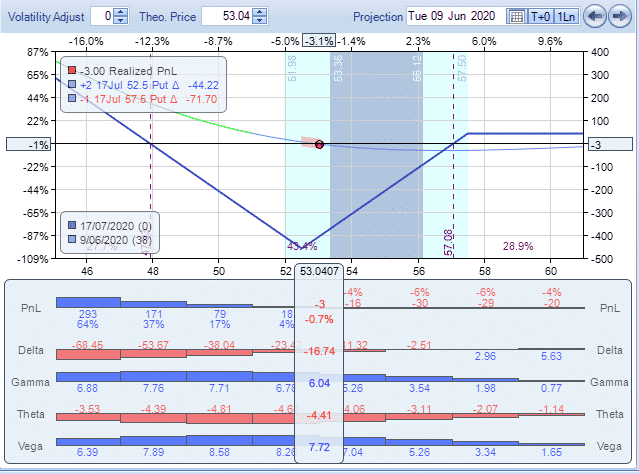After nine days, on 18 of June, the stock price has dropped to \$45, this value is already below the lower breakeven point, the trade has made \$309.

Delta has a negative value of 69.09.

We decided to close the trade.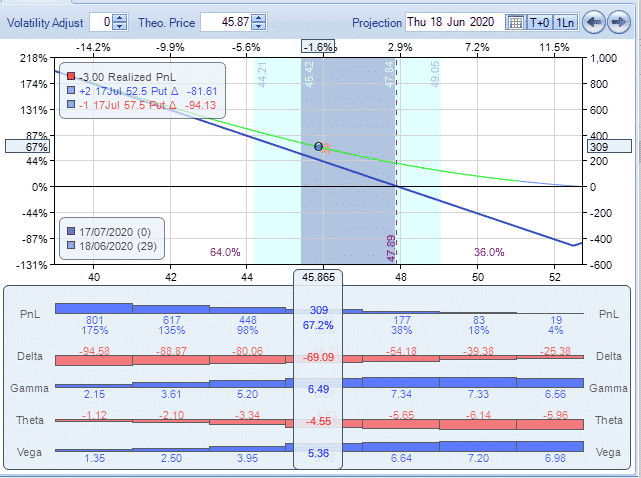The maximum possible loss of the put spread was \$500 -\$41 =  \$459,  the trade made a profit of 67%.

## Summary

Put ratio backspreads are trading strategies that you can consider when you expect the stock will move downside and you want protection on the upside.

For this reason, they should only be placed on stocks the trader thinks will move lower.

A good time to enter this type of trade is at the end of a rally when it is expected a big downside move.

One nice feature of the trade is that there is very little or no risk on the upside and sometimes, even the ability to generate a small amount of premium if the stock rallies.